## ML Aggarwal Class 9 Solutions for ICSE Maths Chapter 2 Compound Interest Chapter Test

Question 1.
₹ 10000 were lent for one year at 10% per annum. By how much more will the interest be, if the sum was lent at 10% per annum, interest being compounded half-yearly ?
Solution:
Principal = ₹10000
Rate of interest (r) = 10% p.a.
Period = 1 year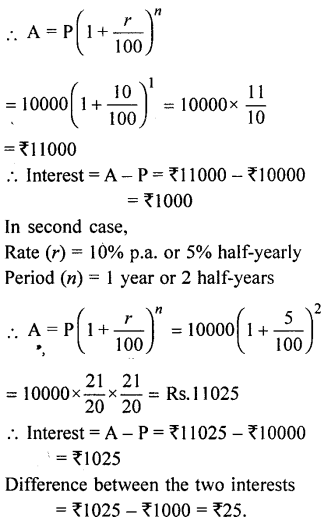Question 2.
A man invests ₹3072 for two years at compound interest. After one year the money amounts to ₹3264. Find the rate of interest and the amount due at the end of 2nd year.
Solution:
Principal (P) = ₹3072
Amount (A) = ₹3264
Period (n) = 1 year
We know that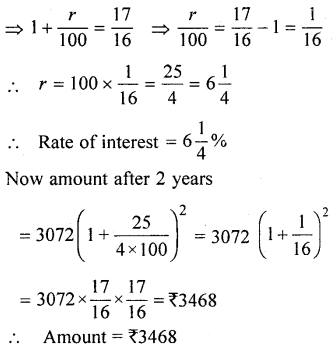Question 3.
What sum will amount to ₹28090 in two years at 6% per annum compound interest ? Also find the compound interest.
Solution:
Amount (A) = ₹28090
Rate (r) = 6% p.a.
Period (n) = 2 year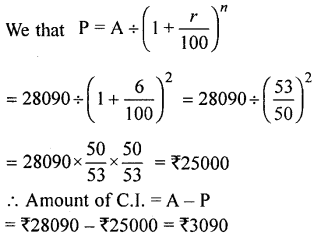Question 4.
Two equal sums were lent at 5% and 6% per annum compound interest for 2 years. If the difference in the compound interest was ₹422 find :
(i) the equal sums
(ii) compound interest for each sum.
Solution:
Let each equal sum = ₹100
In first case, rate (r) = 5%
Period (n) = 2 years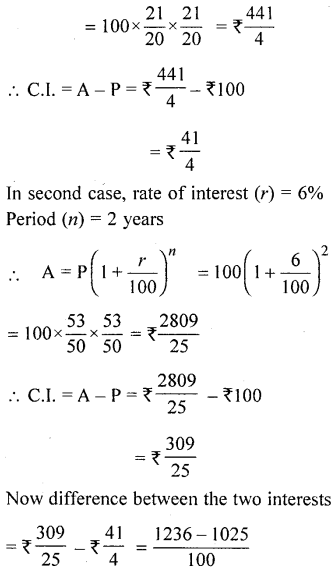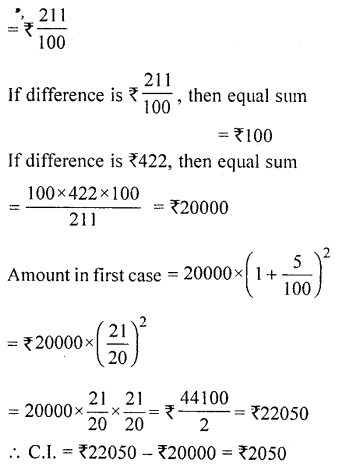Question 5.
The compound interest on a certain sum of money for 2 years is ₹1331·20 and the simple interest on the same sum for the same period and at the same rate is ₹1280. Find the sum and the rate of interest per annum.
Solution:
C.I. for 2 years = ₹1331·20
and S.l. for 2 years = ₹1280·00
Difference = ₹1331·20 – ₹1280·00
= ₹51·20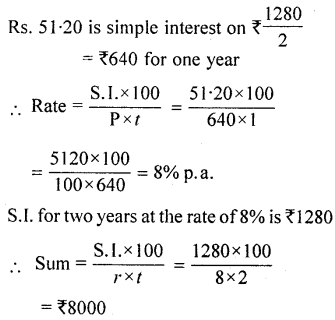Question 6.
On what sum will the difference between the simple and compound interest for 3 years at 10% p.a. is ₹232·50 ?
Solution:
Let sum (P) = ₹100
Rate (r) = 10% p.a.
Period (n) = 3 years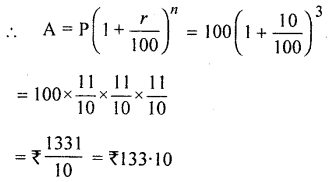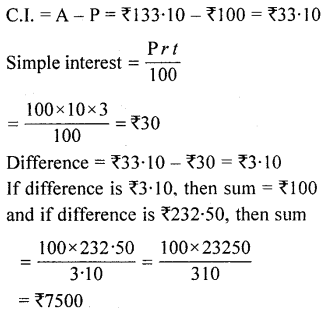Question 7.
The simple interest on a certain sum for 3 years is ₹1080 and the compound interest on the same sum at the same rate for 2 years is ₹741·60. Find
(i) the rate of interest,
(ii) the principal.
Solution:
Simple interest for 3 years = ₹ 1080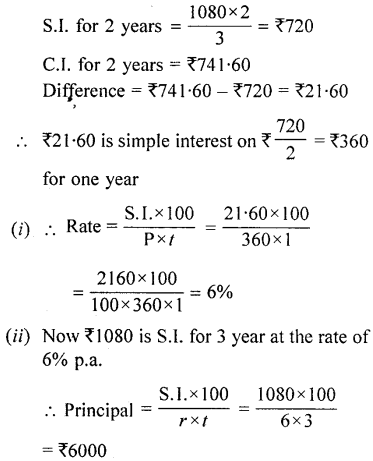Question 8.
In what time will ₹2400 amount to ₹2646 at 10% p.a. compounded semiannually ?
Solution:
Amount (A) = ₹2646
Principal (P) = ₹2400
Rate (r) = 10% p.a. or 5% semi-annually Let period = n half-years
We know that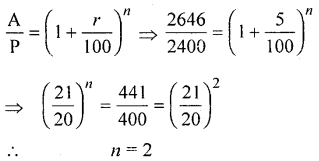Hence time = 2 half-years or 1 year

Question 9.
Sudarshan invested ₹60000 in a finance company and received ₹79860 after $$1 \frac{1}{2}$$ years. Find the rate of interest per annum compounded half-yearly.
Solution:
Principal (P) = ₹60000
Amount (A) = ₹79860
Period (n) = $$1 \frac{1}{2}$$years = 3 half-years
We know thatQuestion 10.
The population of a city is 320000. If the annual birth rate is 9·2% and the annual death rate is 1·7%, calculate the population of the town after 3 years.
Solution:
Birth rate = 9·2%
and Death rate = 1·7%
∴ Net growth rate = 9·2 – 1·7 = 7·5%
Present population (P) = 320000
Period (n) = 3 years
∴ Population after 3 years (A)Question 11.
The cost of a car purchased 2 years ago, depriciates at the rate of 20% every year. If its present worth is ₹315600, find
(i) its purchased price
(ii) its value after 3 years.
Solution:
Present value of car = ₹315600
Rate of depreciation (r) = 20%Question 12.
Amar Singh started a business with an initial investment of ₹400000. In the first year, he incurred a loss of 4%. However, during the second year, he earned a profit of 5% which in the third year rose to 10%. Calculate his net profit for the entire period of 3 years.
Solution:
Investment (P) = ₹400000
Loss in the first year = 4%
Profit in the second year = 5%
Profit in the third year = 10%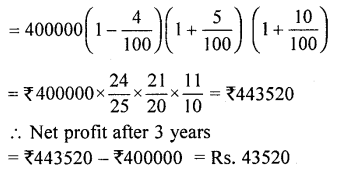Question 13.
The cost of a washing machine depreciates by ₹720 during the second year and by ₹648 during the third year. Calculate :
(i) the rate of depreciation per annum.
(ii) the original cost of the machine.
(iii) the value of the machine at the end of third year.
Solution:
Amount of depreciation for the second year = ₹720
and amount of depreciation for third year = ₹648
Difference = ₹720 – ₹648 = ₹72
∴ ₹72 is depreciation on ₹720 for 1 year.